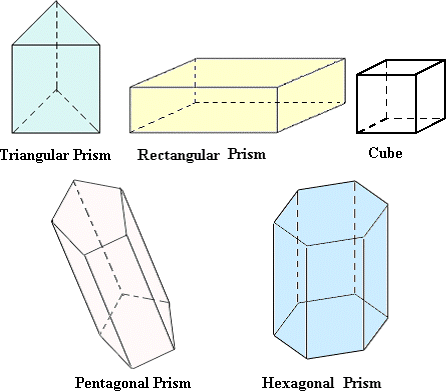Definition Of Prism

A prism is a polyhedron consisting of two parallel, congruent faces called bases.

A prism is named by the shape of its base.
Triangular Prism: A Prism with triangular bases is a Triangular Prism.
Rectangular Prism: A Prism with rectangular bases is a Rectangular Prism.
Cube: A prism with square bases is a cube. All six faces of a cube are squares.
Pentagonal Prism: A Prism with pentagonal bases is a Pentagonal Prism.
Hexagonal Prism: A Prism with hexagonal bases is a Hexagonal Prism.
Octagonal Prism: A Prism with octagonal bases is an Octagonal Prism.

Example of Prism

The following figure shows a few prisms.Solved Example on Prism

Ques: An octagonal prism has ____.

Choices:

A. one vertex
B. eight congruent faces and one base
C. six congruent lateral faces
D. eight lateral faces that are congruent